## The Difference Between Truth and Facts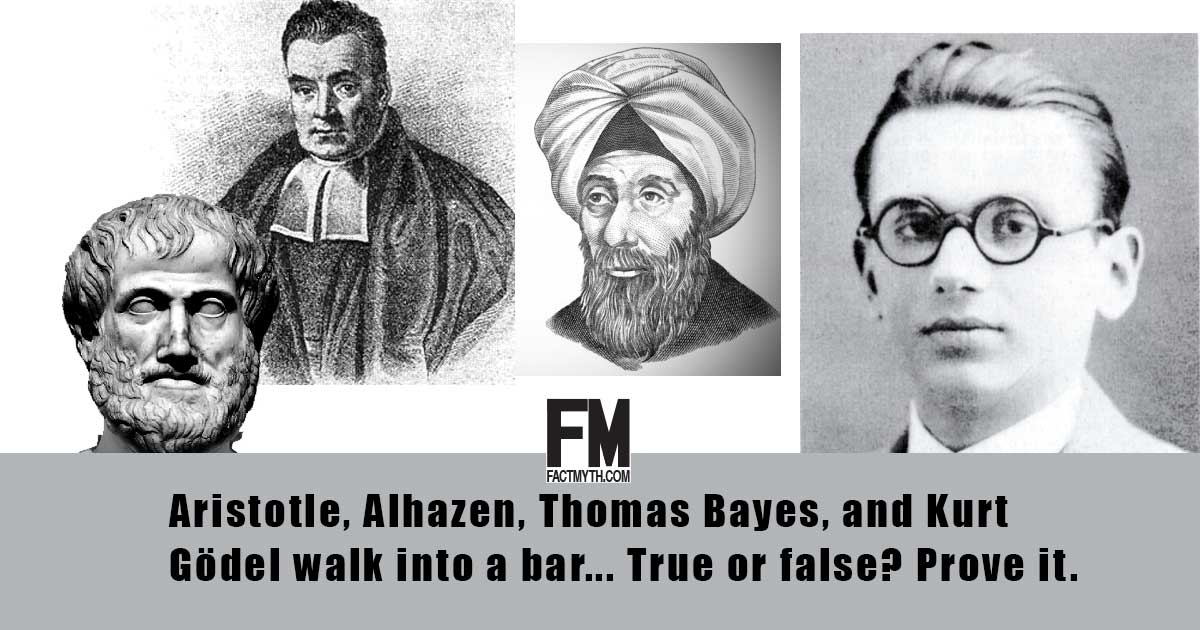Truth and facts have a lot in common, but they are not exactly the same. Truth is something that is the case. Facts are true statements. Truth is best described using facts and logical reasoning.

## What is a Truth-Value?A truth-value is a label that is given to a statement (a proposition) that denotes the relation of the statement to truth.

## There is No Such Thing as Objective TruthThe idea that all truth is subjective, that there is no objective truth, is a myth. Everything either has an absolute truth value (even if we can’t know it) or is an opinion or belief.

## If You Tell the Truth, You Don’t Have to Remember Anything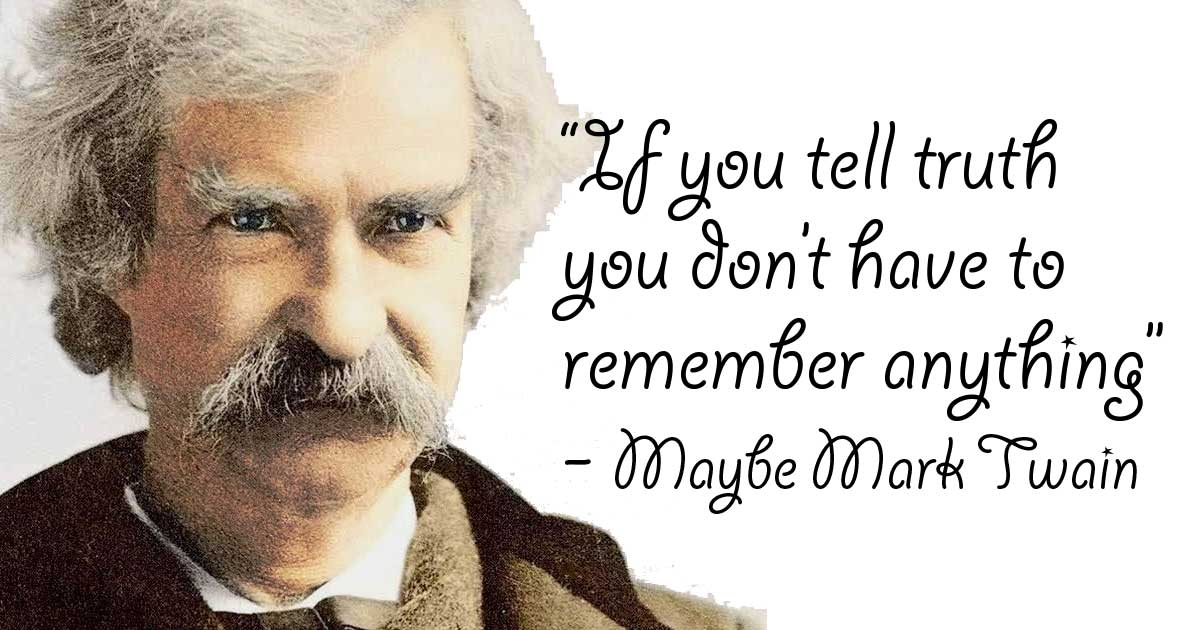Mark Twain is said to have said, “If you tell the truth you don’t have to remember anything”, meaning if you tell a lie you have to remember what lie you told to who.

## The Nature of Truth and the Different Types of Truth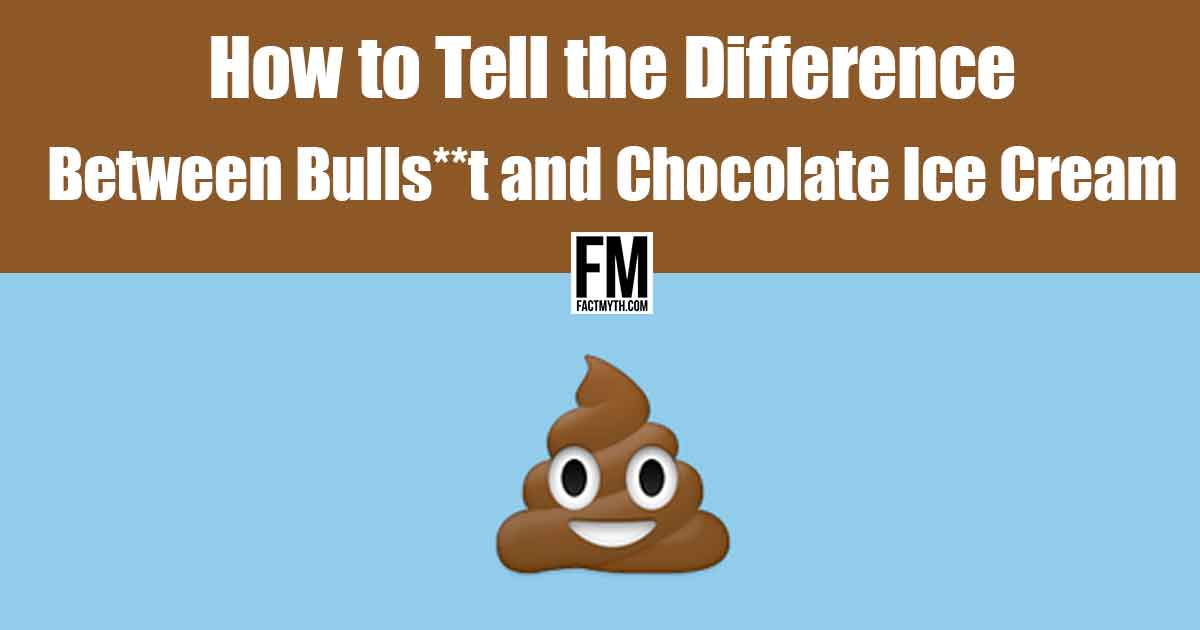We explore the nature of truth, the different types of truth, and the different types of entities who report truth to better understand the nature of information.

## Bayes’ Theorem Can Calculate Probable Truth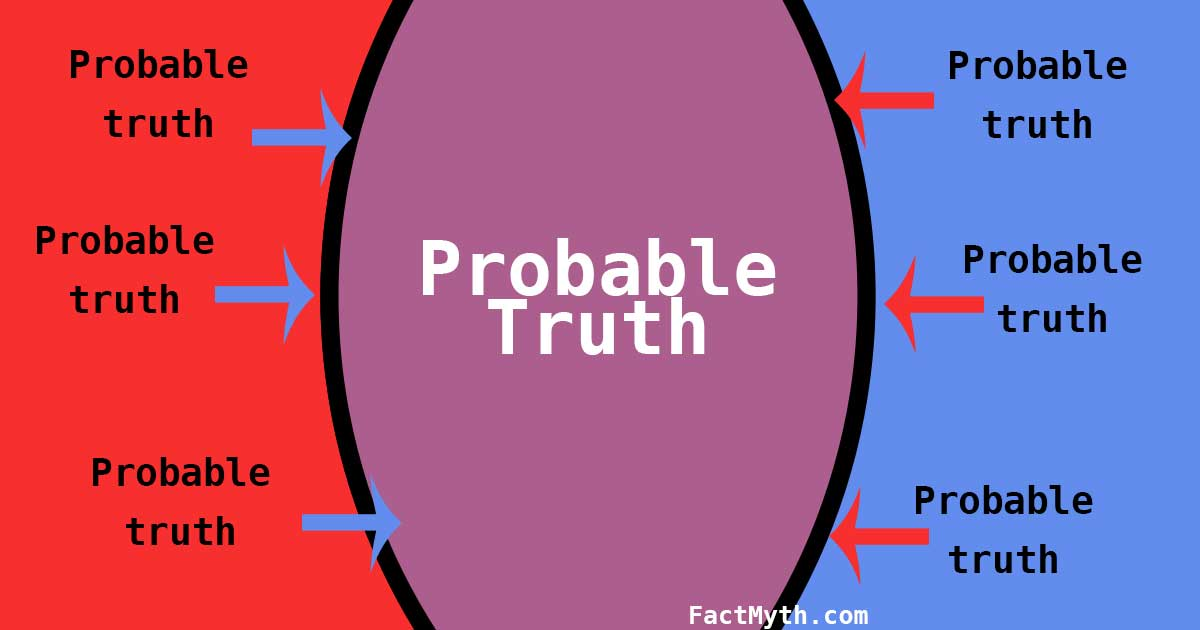Bayes’ theorem is a probability theory used to calculate the likelihood of an event being true or not true based on conditions related to the event. (i.e. an equation used for calculating conditional probabilities).

## Inductive Reasoning ExplainedWe explain inductive reasoning, a bottom-up reasoning method that reasons by consistency, comparing particulars and probabilities to find likely truths.

## You Can’t Prove a Negative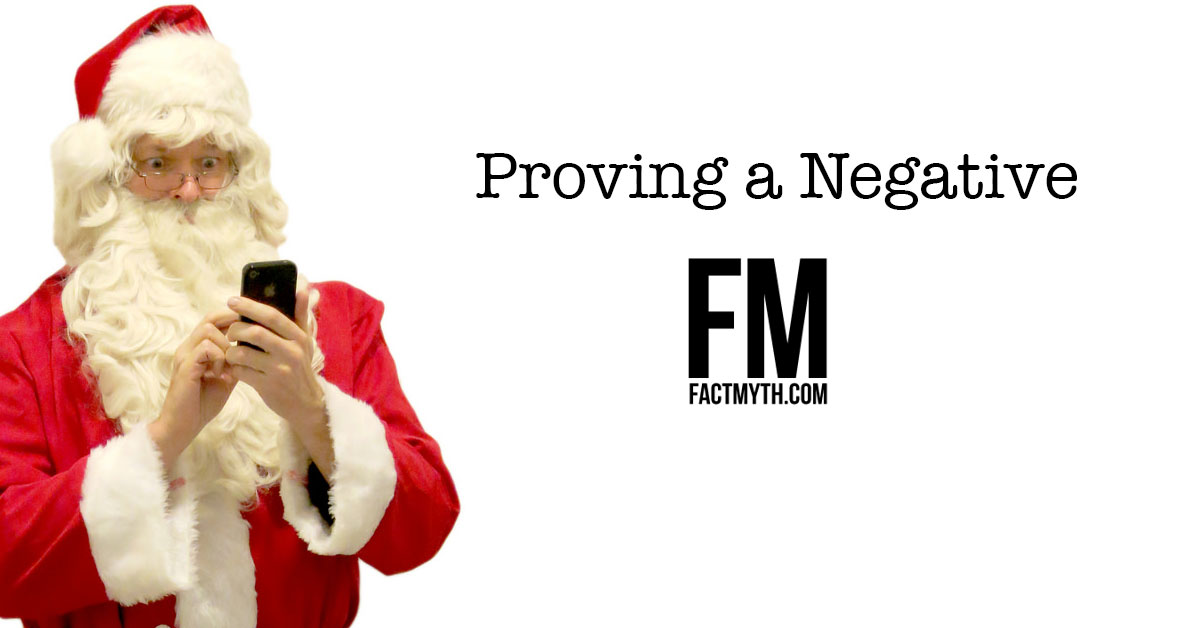The saying “you can’t prove a negative” isn’t accurate. Proving negatives is a foundational aspect of logic (ex. the law of contradiction).

## The Different Types of Reasoning Methods Explained and Compared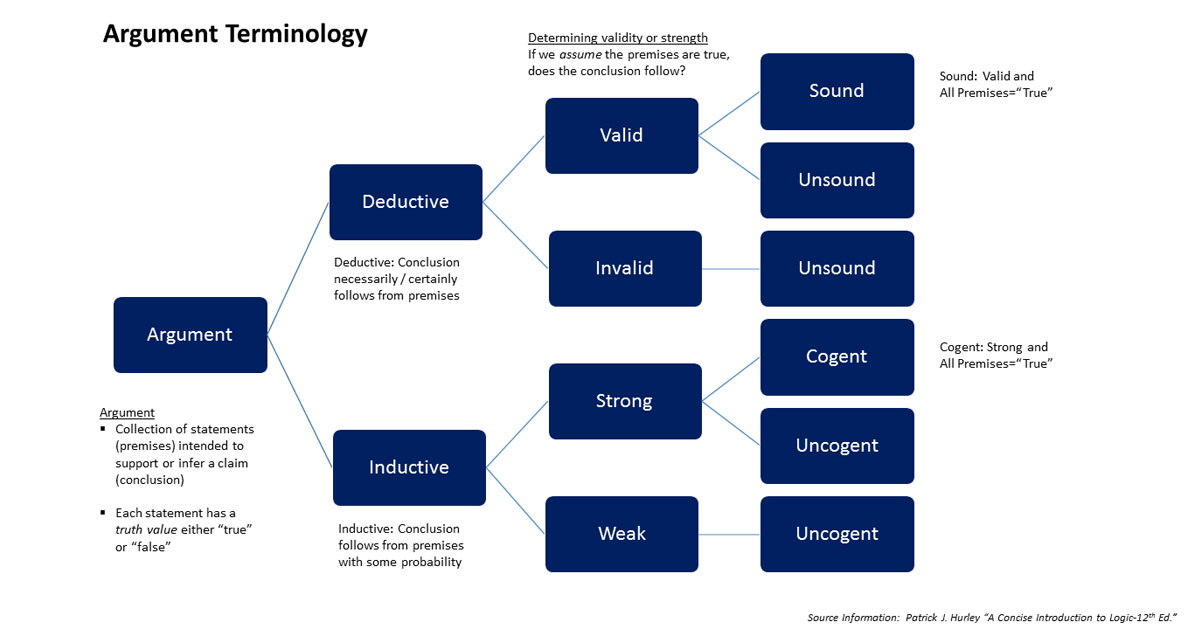We explain and compare the different types of reasoning methods including deductive, inductive, abductive, analogical, and fallacious reasoning.

## The Basics of a Theory of Knowledge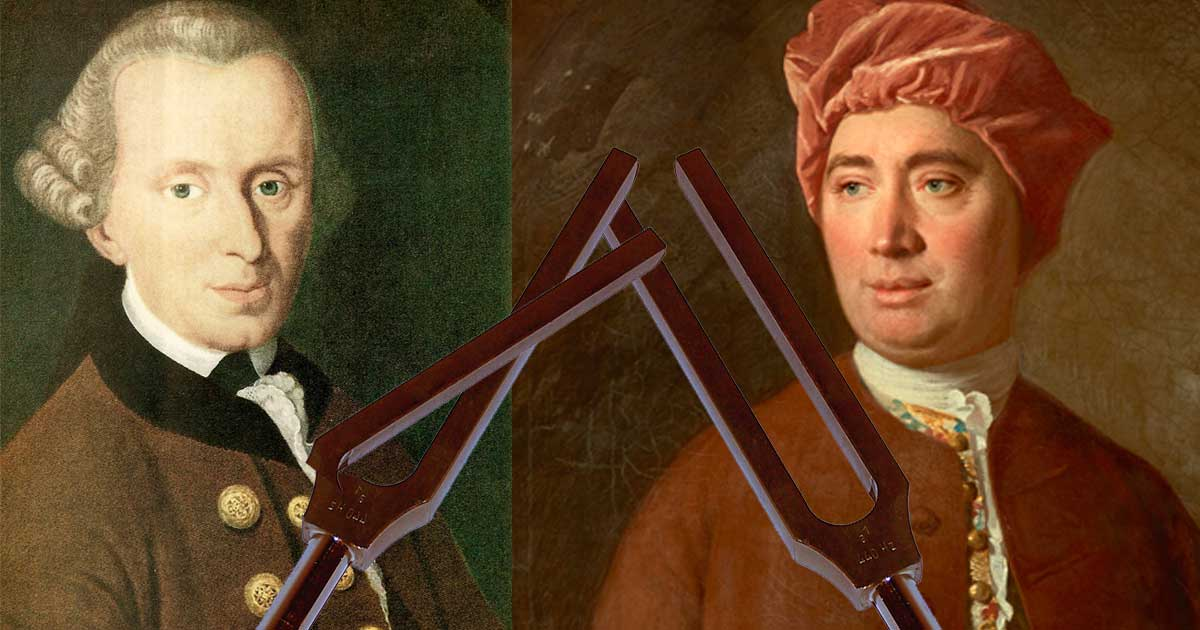We present a basic theory of human knowledge to help illustrate some essentials of “what we can know” and “how we can know it.”